Example Questions

Example Question #1220 : Act Science

A student was interested in determining the relationship between the current, voltage, and resistance in a direct circuit, such as those exemplified by batteries connected to light bulbs. The student built the circuit presented in Figure 1 using a 2 ohm resistor.

Figure 1: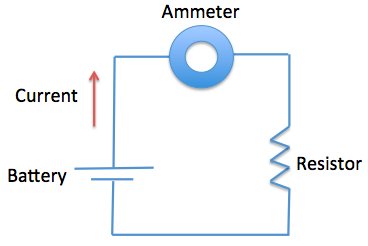The current that flows through the circuit can be calculated using the equation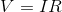, whereis the voltage of the battery,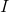is the current flowing through the circuit, andis the resistance of the resistor.

The student used a 2 ohm resistor and batteries of various voltages to obtain the results in Table 1. The currents shown in the table are NOT calculated using the formula, but instead directly measured from the circuit using an ammeter. It is important to note that the measured current will only exactly equal the calculated current if the system contains no internal resistance.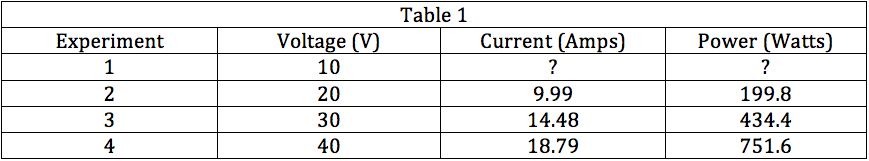Based on Table 1, the power of a circuit can be calculated using the equation __________.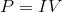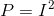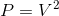Explanation:

We can use the values provided in Table 1 to determine how power is being calculated. Using Experiment 2 as a guide, we can see that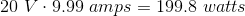, or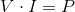. The same relationship holds for all other experiments, so we can assume the equation to calculate power is.

Example Question #31 : How To Find Synthesis Of Data In Physics

A scientist is exploring the nature of energy and work in two experimental systems.

Experiment 1

She first sets up a system with a ball on an inclined plane, and calculates the potential and kinetic energies of the ball at three positions. She places the ball at position 1, stops the ball after rolling to position 2, and then again allows it to roll to position 3. The measured kinetic and potential energies are shown in the table, along with measurements of the force of friction acting on the ball.

 Ball Position Potential Energy Kinetic Energy Force of Friction 1 150 kJ X kJ 0 N 2 75 kJ 75 kJ 0 N 3 X kJ 150 kJ 0 N

Experiment 2

The same scientist then sets up another experiment with the same ball, again at three positions.  The ball is provided a slight push, and then allowed to roll down three levels without any additional external input of energy.

She uses the following formulae to calculate the energy levels of the ball:

Potential Energy = Mass of the Ball x Acceleration Due to Gravity x Height of the Ball

Kinetic Energy = (1/2) x Mass of the Ball x (Velocity of the Ball)2

She also measures the internal energy of the ball, a value that she defines as the amount of energy contained in the motion of the molecules that make up the matter of the ball.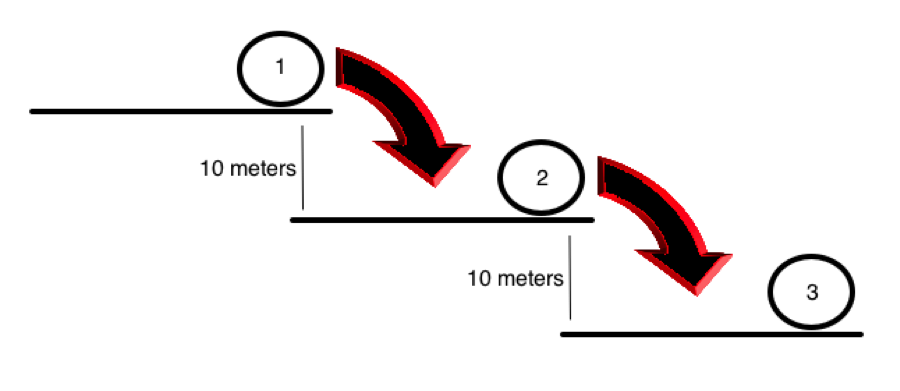Position Potential Energy Velocity Internal Energy 1 A 1 m/s 5 kJ 2 B 5.47 m/s 6 kJ 3 C 10.94 m/s 7 kJ

In Experiment 1, the experimenter changes the material on the surface of the ball and the surface of the ramp. She finds that the force of friction on the ball is now equal to 5 N at points 1, 2, and 3. Which of the following is most likely true?

I.  Force of friction is dependent on the nature of the surfaces in contact

II.  Force of friction is inversely proportional to potential energy, and dependent on the nature of the surfaces in contact

III.  Force of friction is inversely proportional to kinetic energy, and dependent on the nature of the surfaces in contact

III only

I and II

I and III

II only

I only

I only

Explanation:

According to the table in the first experiment, friction does not change with potential or kinetic energy. However, we know that it does change with the nature of the surfaces in contact, as the question tells us this much. Therefore, it must be dependent on this variable, and not the potential or kinetic energy values.

Example Question #32 : How To Find Synthesis Of Data In Physics

A scientist is exploring the nature of energy and work in two experimental systems.

Experiment 1

She first sets up a system with a ball on an inclined plane, and calculates the potential and kinetic energies of the ball at three positions. She places the ball at position 1, stops the ball after rolling to position 2, and then again allows it to roll to position 3. The measured kinetic and potential energies are shown in the table, along with measurements of the force of friction acting on the ball.

 Ball Position Potential Energy Kinetic Energy Force of Friction 1 150 kJ X kJ 0 N 2 75 kJ 75 kJ 0 N 3 X kJ 150 kJ 0 N

Experiment 2

The same scientist then sets up another experiment with the same ball, again at three positions.  The ball is provided a slight push, and then allowed to roll down three levels without any additional external input of energy.

She uses the following formulae to calculate the energy levels of the ball:

Potential Energy = Mass of the Ball x Acceleration Due to Gravity x Height of the Ball

Kinetic Energy = (1/2) x Mass of the Ball x (Velocity of the Ball)2

She also measures the internal energy of the ball, a value that she defines as the amount of energy contained in the motion of the molecules that make up the matter of the ball.Position Potential Energy Velocity Internal Energy 1 A 1 m/s 5 kJ 2 B 5.47 m/s 6 kJ 3 C 10.94 m/s 7 kJ

The Law of Conservation of Energy states that energy in the universe cannot be created or destroyed. Which of the following is most likely true in Experiment 1 as the ball rolls from position 1 to position 3?

Total frictional energy is conserved

Kinetic energy is turned into potential energy

Total kinetic energy is conserved

Potential energy is turned into kinetic energy

Total potential energy is conserved

Potential energy is turned into kinetic energy

Explanation:

While energy cannot be created or destroyed, the table in Experiment 1 clearly shows that energy is changing forms.

Example Question #33 : How To Find Synthesis Of Data In Physics

A scientist is exploring the nature of energy and work in two experimental systems.

Experiment 1

She first sets up a system with a ball on an inclined plane, and calculates the potential and kinetic energies of the ball at three positions. She places the ball at position 1, stops the ball after rolling to position 2, and then again allows it to roll to position 3. The measured kinetic and potential energies are shown in the table, along with measurements of the force of friction acting on the ball.

 Ball Position Potential Energy Kinetic Energy Force of Friction 1 150 kJ X kJ 0 N 2 75 kJ 75 kJ 0 N 3 X kJ 150 kJ 0 N

Experiment 2

The same scientist then sets up another experiment with the same ball, again at three positions.  The ball is provided a slight push, and then allowed to roll down three levels without any additional external input of energy.

She uses the following formulae to calculate the energy levels of the ball:

Potential Energy = Mass of the Ball x Acceleration Due to Gravity x Height of the Ball

Kinetic Energy = (1/2) x Mass of the Ball x (Velocity of the Ball)2

She also measures the internal energy of the ball, a value that she defines as the amount of energy contained in the motion of the molecules that make up the matter of the ball.Position Potential Energy Velocity Internal Energy 1 A 1 m/s 5 kJ 2 B 5.47 m/s 6 kJ 3 C 10.94 m/s 7 kJ

The work-energy theorem states that the work done on an object is equal to that object's change in kinetic energy: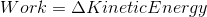As the ball rolls from position 1 to position 2 in Experiment 1, which of the following is most likely true?

Heat is performing most of the work on the ball

We cannot predict the force performing most of the work on the ball

Electric current is performing most of the work on the ball

Friction is performing most of the work on the ball

Gravity is performing most of the work on the ball

Gravity is performing most of the work on the ball

Explanation:

We know that the ball is experiencing an increase in kinetic energy as it rolls down the ramp under the force of gravity. It is most likely, then, that gravity is performing the majority of the work on the ball, as we know that the change in kinetic energy is equivalent to the work being done.

Example Question #34 : How To Find Synthesis Of Data In Physics

The graph below depicts the position of three different cars over a 15-second time interval.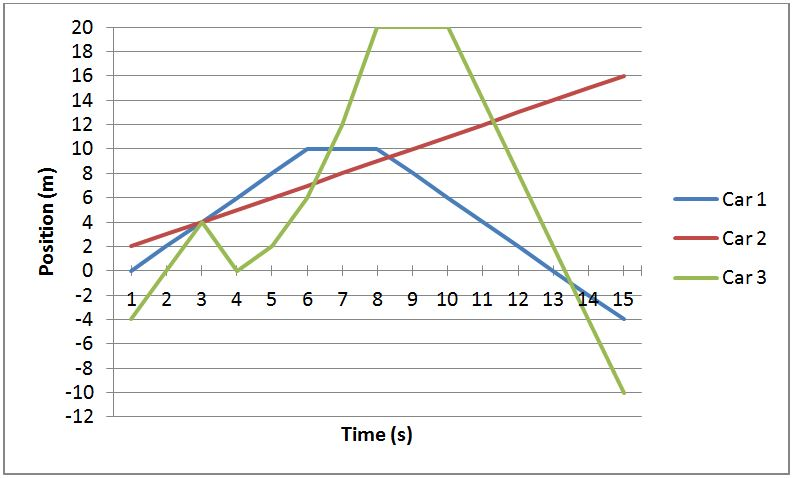Which of the following describes the correct sequence of speed changes of Car 3?

constant, decreasing, increasing, constant, decreasing

constant, zero, constant

constant, increasing, zero, decreasing

constant, increasing, zero, constant

increasing, decreasing, increasing, constant, decreasing

constant, increasing, zero, constant

Explanation:

This question asks you to interpret how the green slope changes. From t = 1 to 4s, the speed of the car is constant (it has a linear slope). From t = 4 to 8 s, the slope becomes greater and greater, indicating that Car 3 is accelerating. From t = 8 s to 10s, Car 3 has the same position, indicating that Car 3 is not moving. Finally, from 10s and onward the slope of Car 3 is constant (neither accelerating or decelerating). Thus, the correct sequence is constant, increasing, zero, constant.

Example Question #35 : How To Find Synthesis Of Data In Physics

Black-body radiation is the energy that is released as light from any object with a non-zero temperature (0 Kelvin, or about –273.15 degrees Celcius). An everyday example of black-body radiation is an electric stovetop. When an electric stove is at its highest setting, energy is pumped into the stovetop, and some of the energy will be released as light, causing it to glow orange. This is black-body radiation at the wavelength of light that corresponds with the color orange.

The visible spectrum of light wavelengths is from about 400 nanometers (high energy) to about 750 nanometers (low energy). The wavelength of light is correlated with the energy of that light, while the intensity refers to the amount of light. For example, a stovetop with very bright orange color does not have different energy per photon of light than a stovetop with a dim orange, as long as they are the same shade. Rather, the brighter stovetop just emits more photons.

The wavelength of black-body radiation of an object can be described by the following equation: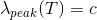, where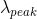is the most prominent (peak) wavelength of the radiation emitted from an object,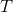is temperature in Kelvin (Celcius plus 273.15), andis a constant.

At a temperature of 100 degrees Kelvin, it has a peak wavelength of 450 nanometers. What would happen if this object were heated by 50 degrees Kelvin?

The object's black-body radiation would change color

The object's black-body radiation would shift from visible to non-visible

The object's black-body radiation would shift from non-visible to visible

The object's black-body radiation would increase in intensity

The object's black-body radiation would decrease in intensity

The object's black-body radiation would shift from visible to non-visible

Explanation:

The answer is that it would go from being visible to not being visible. As we see in the equation, increasing an object's temperature decreases the wavelength of its black-body radiation. We do not know how this temperature increase will affect the intensity of the light. What we do know is that it will lower the black-body radiation wavelength by a factor of 1.5. This would take the wavelength below 400 nanometers, making it fall outside of the visible spectrum as described in the passage.

Example Question #36 : How To Find Synthesis Of Data In Physics

Black-body radiation is the energy that is released as light from any object with a non-zero temperature (0 Kelvin, or about –273.15 degrees Celcius). An everyday example of black-body radiation is an electric stovetop. When an electric stove is at its highest setting, energy is pumped into the stovetop, and some of the energy will be released as light, causing it to glow orange. This is black-body radiation at the wavelength of light that corresponds with the color orange.

The visible spectrum of light wavelengths is from about 400 nanometers (high energy) to about 750 nanometers (low energy). The wavelength of light is correlated with the energy of that light, while the intensity refers to the amount of light. For example, a stovetop with very bright orange color does not have different energy per photon of light than a stovetop with a dim orange, as long as they are the same shade. Rather, the brighter stovetop just emits more photons.

The wavelength of black-body radiation of an object can be described by the following equation:, whereis the most prominent (peak) wavelength of the radiation emitted from an object,is temperature in Kelvin (Celcius plus 273.15), andis a constant.

What would happen to the peak wavelength of an object's black-body radiation if its temperature (in Kelvin) were doubled?

The peak wavelength would increase by a factor of 4.

The peak wavelength would increase by a factor of 2.

The peak wavelength would remain constant.

The peak wavelength would decease by a factor of 4.

The peak wavelength would decrease by a factor of 2.

The peak wavelength would decrease by a factor of 2.

Explanation:

The correct answer is that it would decrease by a factor of 2. Since the the peak wavelength multiplied by the temperature are set equal to a constant, if one increases, the other has to decrease proportionally. This is a perfect inverse relationship.

Example Question #37 : How To Find Synthesis Of Data In Physics

Black-body radiation is the energy that is released as light from any object with a non-zero temperature (0 Kelvin, or about –273.15 degrees Celcius). An everyday example of black-body radiation is an electric stovetop. When an electric stove is at its highest setting, energy is pumped into the stovetop, and some of the energy will be released as light, causing it to glow orange. This is black-body radiation at the wavelength of light that corresponds with the color orange.

The visible spectrum of light wavelengths is from about 400 nanometers (high energy) to about 750 nanometers (low energy). The wavelength of light is correlated with the energy of that light, while the intensity refers to the amount of light. For example, a stovetop with very bright orange color does not have different energy per photon of light than a stovetop with a dim orange, as long as they are the same shade. Rather, the brighter stovetop just emits more photons.

A scientist decides to conduct an experiment to see how the temperature of an object affects the total energy released in its black-body radiation. The scientist conducts the experiment at very low temperatures and measures the energy released by the object in units of a hundred-millionth of a joule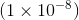. The results are shown below: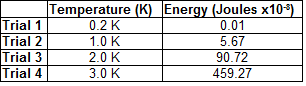Based on the data collected, what could we predict the energy to be for an object at a temperature of 4.0K?

540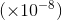Joules

344Joules

612Joules

1450Joules

459Joules

1450Joules

Explanation:

The correct answer is the one that follows the trend shown in the chart. When the temperature was doubled, (from 1K to 2K), the energy increased by a factor of about 16. This means that temperature and energy here are related exponentially. In fact, they are related by an exponent of 4. That is, multiplying one by 2, increases the other by a factor of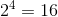Therefore, the answer is approximately 16 times the answer for trial 3. This we can simply estimate (you don't have to actually do the math) and we can rule out any answer that is either lower than 459 or not sufficiently greater than 459.

Example Question #38 : How To Find Synthesis Of Data In Physics

An engineer wants to design a prank toy that will be thrown onto the ground. It looks like a superball (bouncing ball), but does not bounce. The engineer has Subtance A, Substance B, and Substance C that he drops from various heights. All of the tests are performed by dropping the substances on the same surface and each height is tested multiple times. The error between experiments is minimal and too small to be seen on the graph. The engineer records the maximum rebound height of each substance at the various drop heights. This data is displayed in the graph below.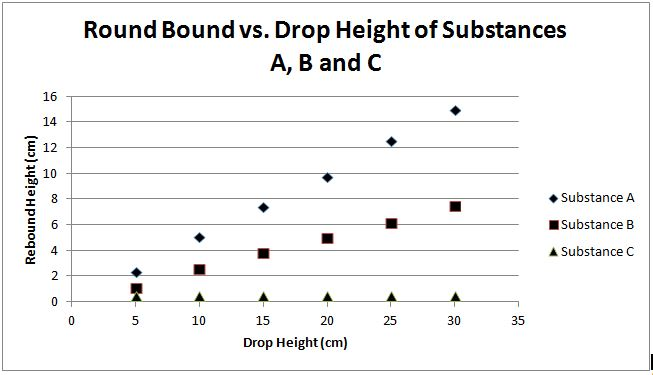Which substance would be best for the engineer to investigate further for developing the prank bouncing ball ?

There is not enough information to answer this question

Substance C

Substance A and Substance B

Substance B

Substance A

Substance C

Explanation:

Substance C is the best material for this task. In the plot, the ball composed of Substance C consistently has the lowest rebound height. Additionally, Substance C's rebound height does not significantly change despite the change in drop height.

Example Question #39 : How To Find Synthesis Of Data In Physics

A researcher is investigating new solar technology. The researcher looks at combinations of solar panels and solar rechargeable batteries that were provided from research labs A, B, C and D. The combinations of solar panels and solar rechargeable batteries are measured for their efficiency, the amount of time it takes to fully charge a battery, and the life of each fully charged battery used to power a home. The experiments were conducted using a UV light. The batteries recharge when the solar panels are exposed to UV light and are depleted of charge when being used for power. The solar panels will not recharge the batteries on cloudy days.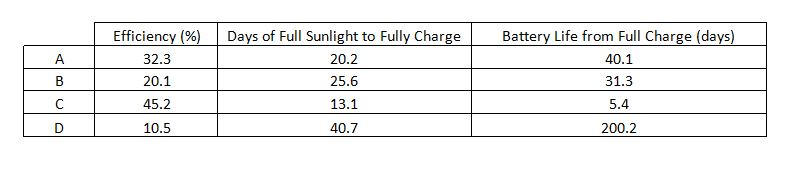An individual lives in an area that will experience 180 days and nights of sunlight in a row (assume no clouds during this time) and the remainder of the year will have no sunlight. What setup should the researcher recommend, if the individual plans on only using solar energy to power his or her home?

B

A

C

D

Cannot be determined